# NCERT Exemplar Solutions for Class 11 Maths Chapter 6 Linear Inequalities

NCERT Exemplar Solutions for Class 11 Maths Chapter 6 Linear Inequalities are provided here for the students to refer to. A linear inequality is a fundamental concept that forms the basis for much more advanced topics. Therefore, one of the best guides to help you understand this concept is NCERT Exemplar Solutions for Class 11 Maths Chapter 6 – Linear Inequalities. It is designed by knowledgeable teachers with years of experience in the relevant field. Therefore, NCERT Exemplar Solutions are the best study resources you can choose for your study needs. We mainly focus on providing answers which are in an easy format to remember, which will help students to clearly understand and remember the formulae.

This chapter of NCERT Exemplar Solutions for Class 11 mainly focuses on the solutions related to inequalities. A linear function with inequalities is called linear inequality. It contains one of the symbols of linear equality. It acts exactly like a linear equation except for the inequality sign, which replaces the equality sign. Some of the linear inequalities of real numbers are mentioned below:

1. Two-dimensional linear equalities
2. Linear inequalities in general dimensions
3. Systems of linear inequalities

Linear programming, along with the polyhedra, are some of the major applications of linear equalities. The PDF of Chapter 6 exemplar solutions can be downloaded easily and can also be used as a reference tool while preparing for final exams. Let us have a look at some of the important concepts that are discussed in this chapter.

• The solution of an inequality
• Representation of solution of a linear inequality in one variable on a number line
• Graphical representation of the solution of a linear inequality
• Two important results on positive real numbers

## Download the PDF of NCERT Exemplar Solutions for Class 11 Maths Chapter 6 Linear Inequalities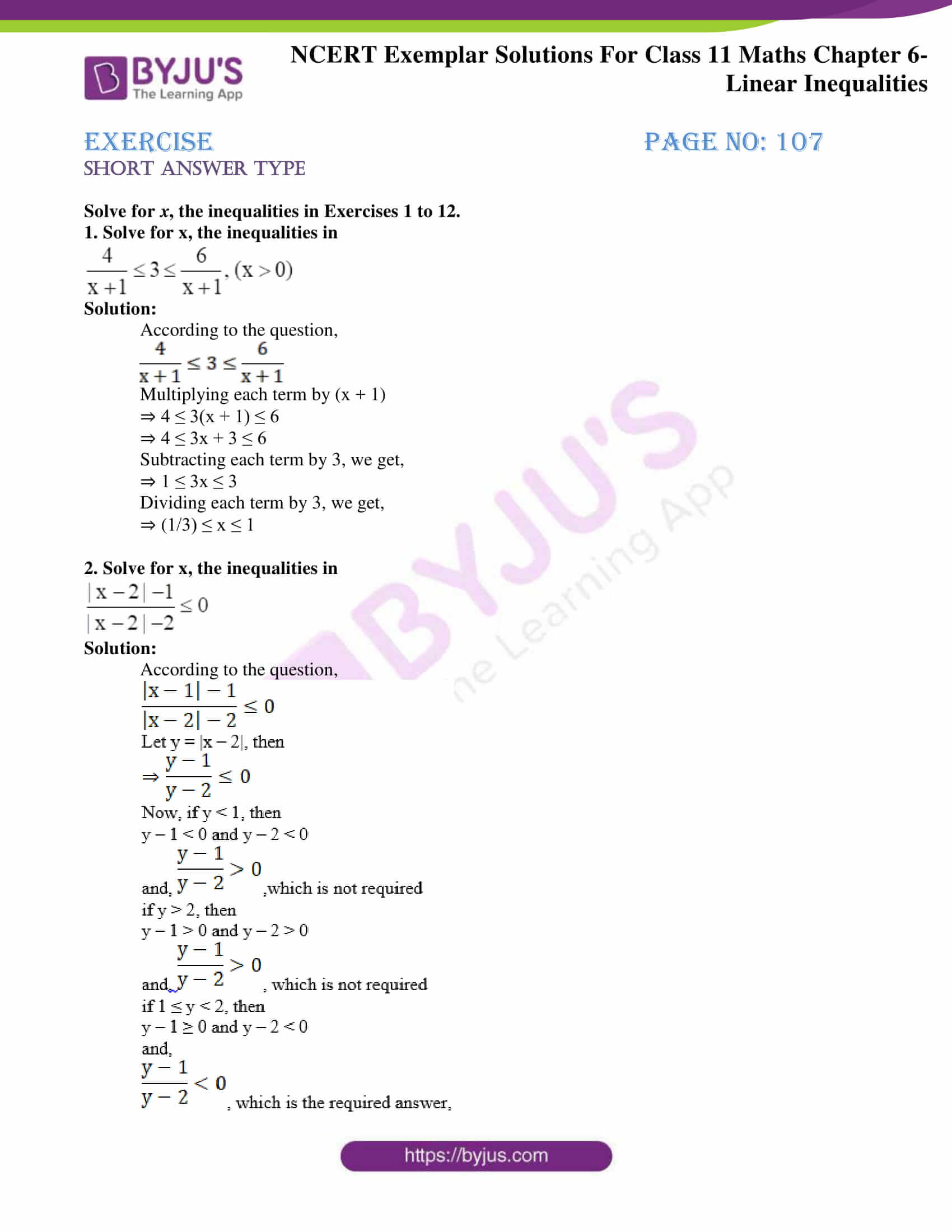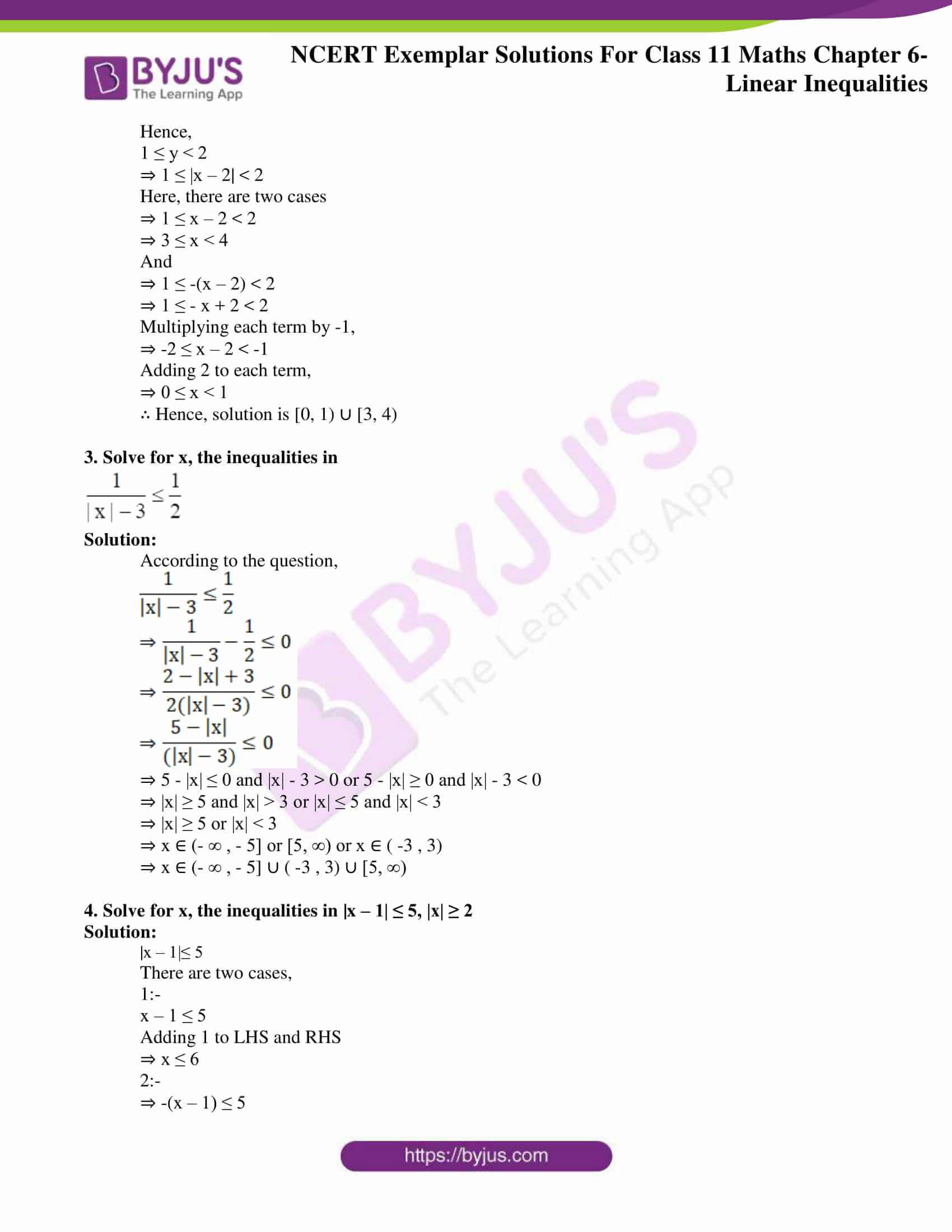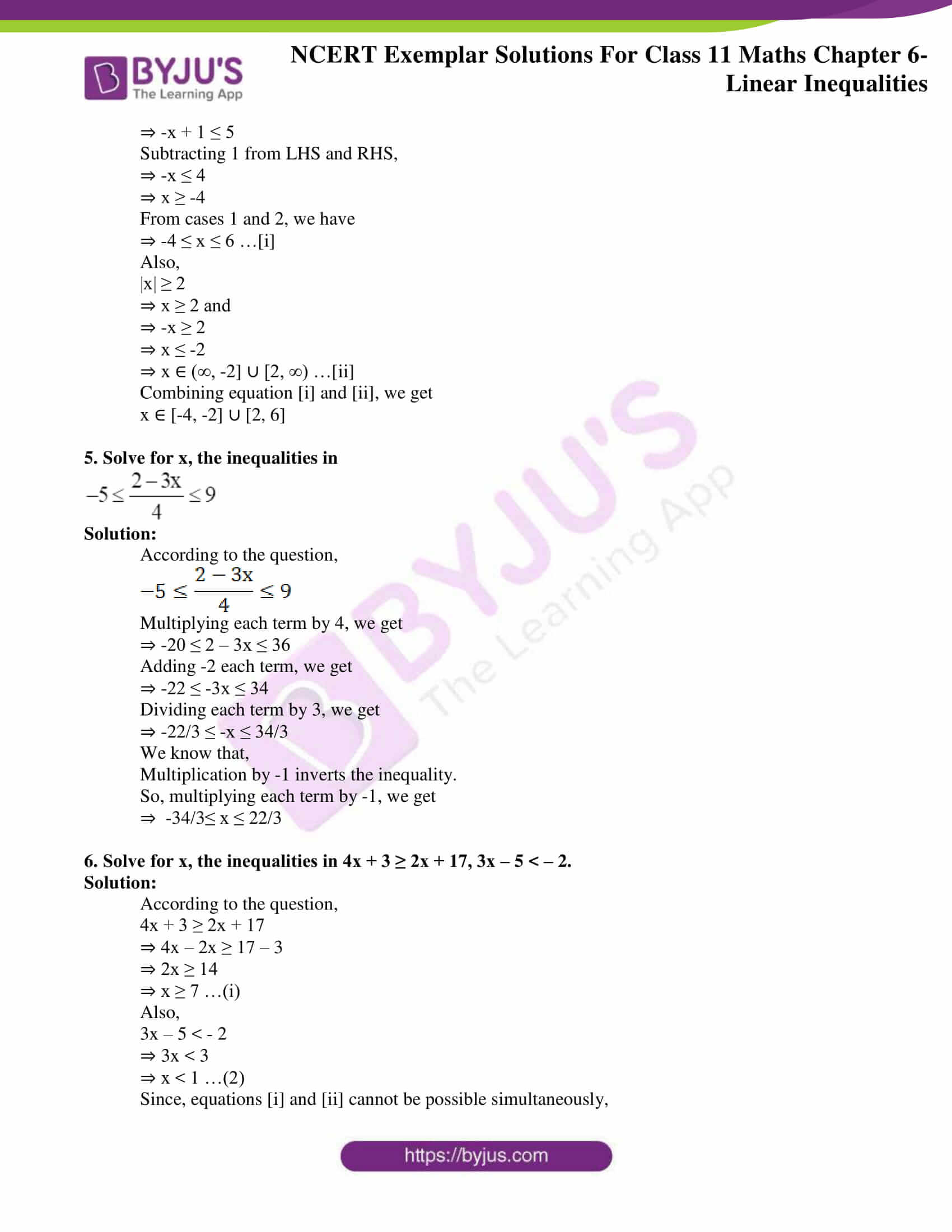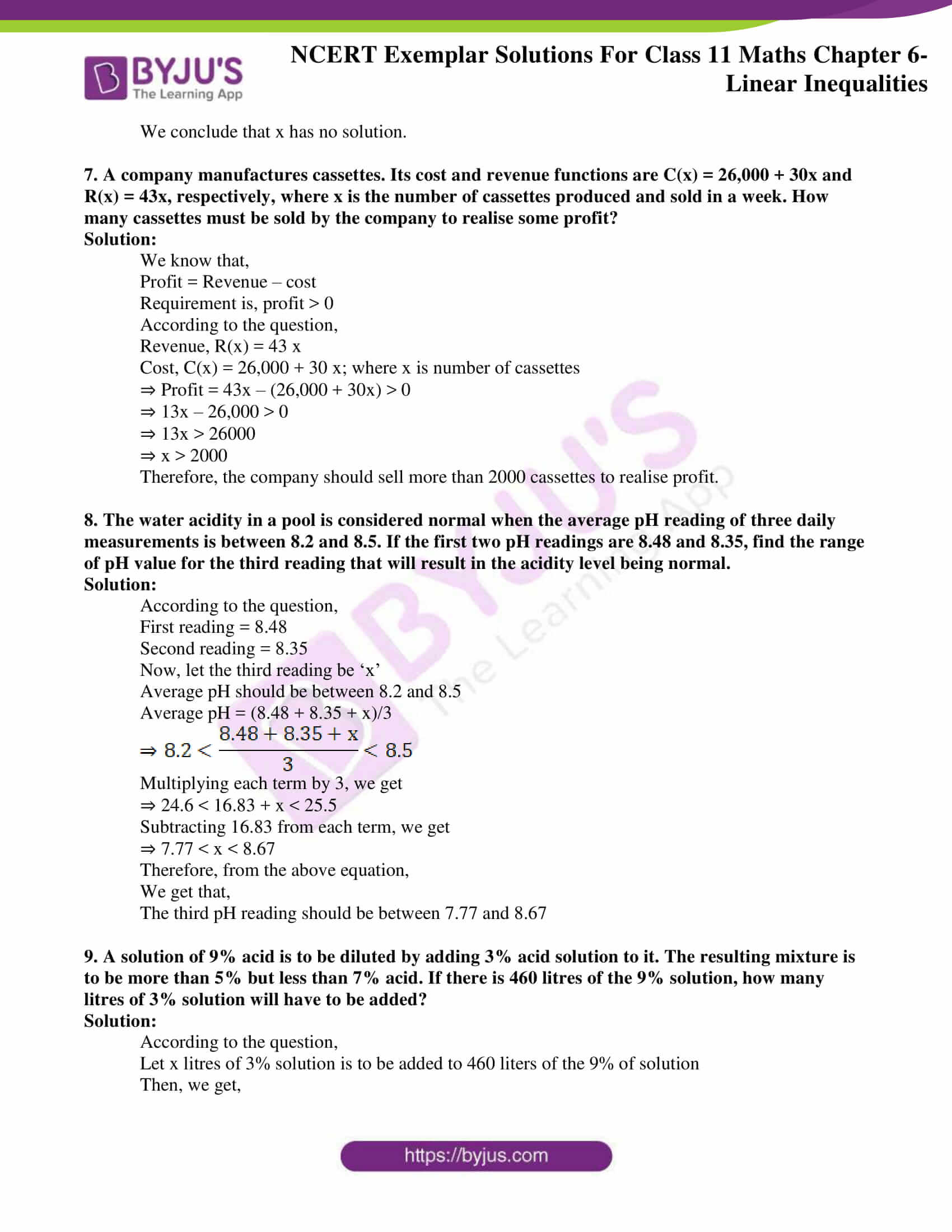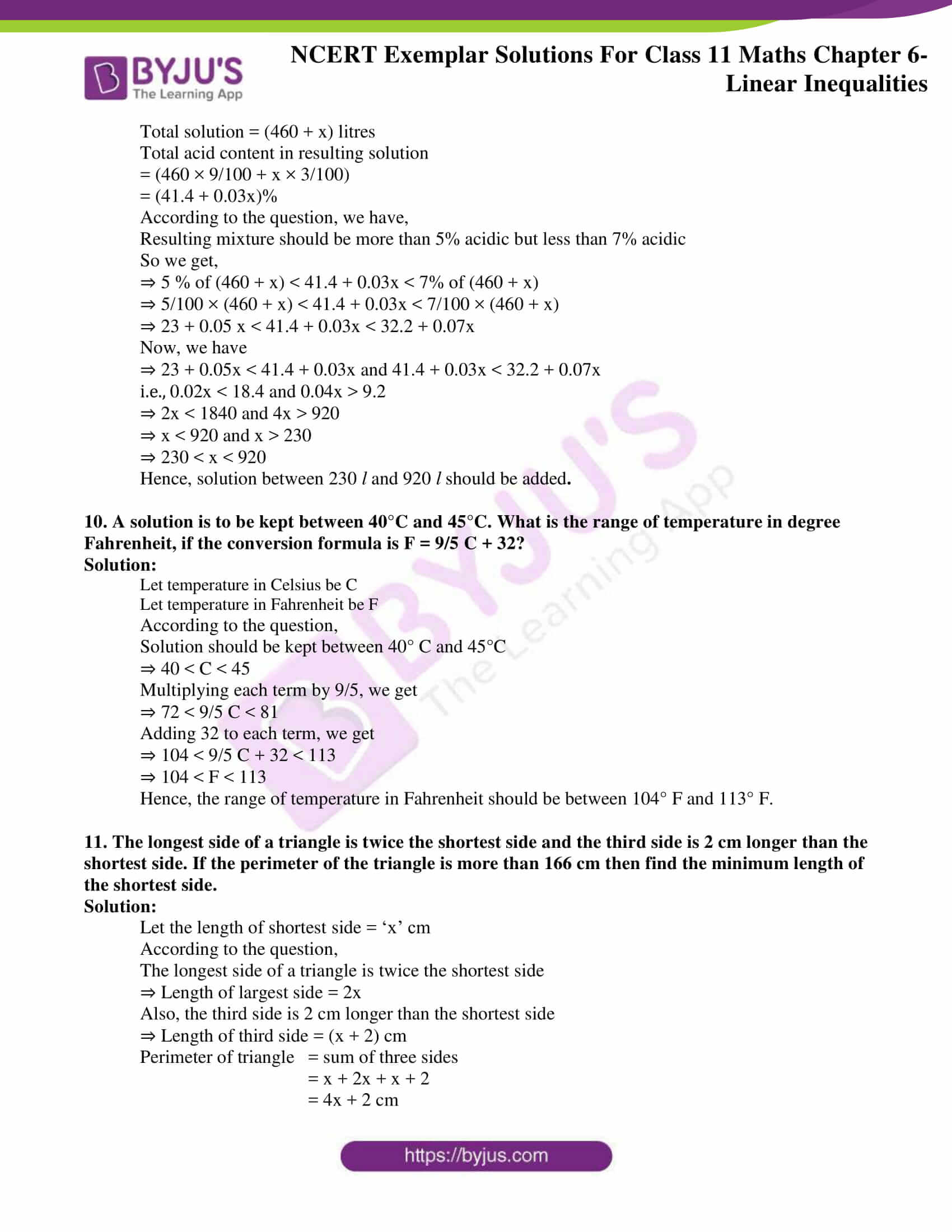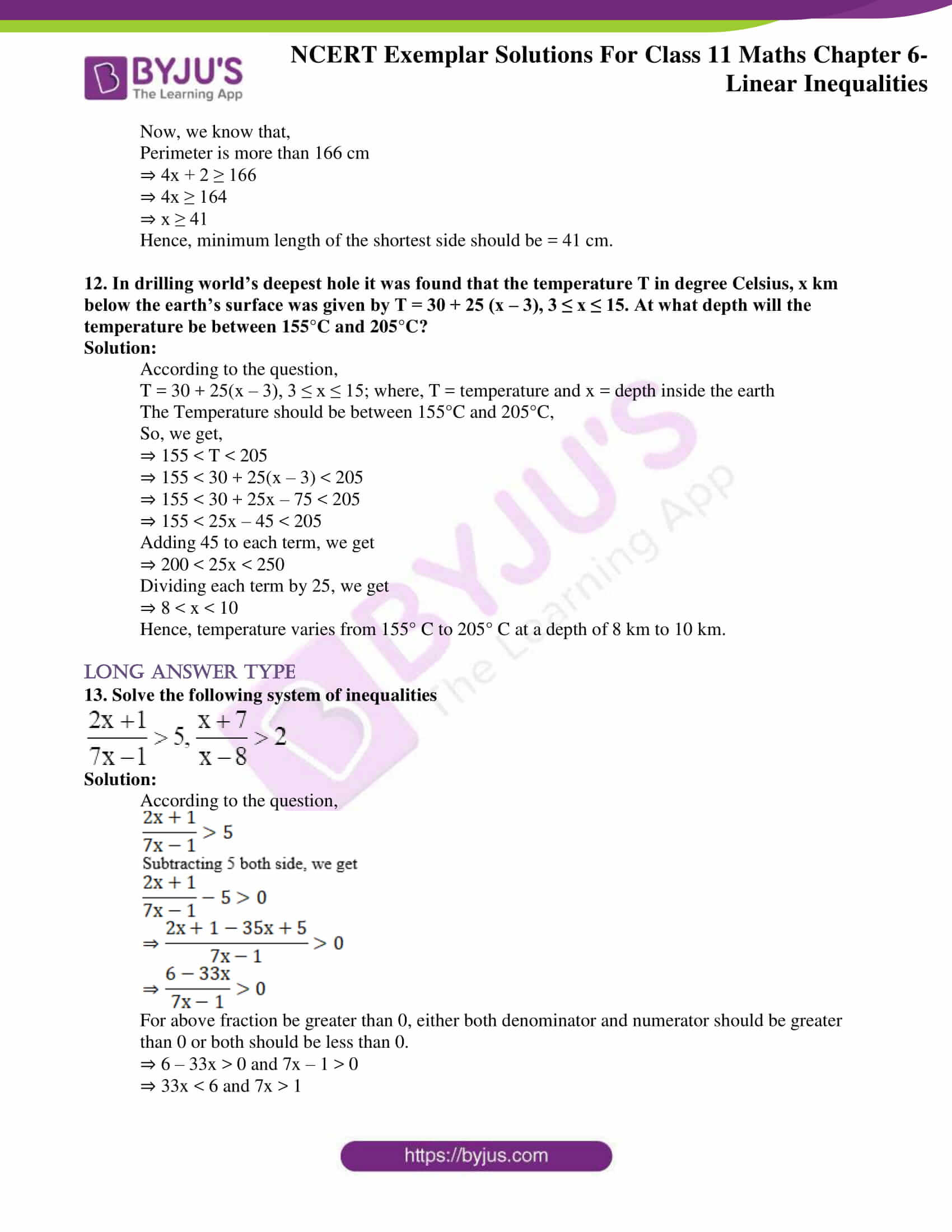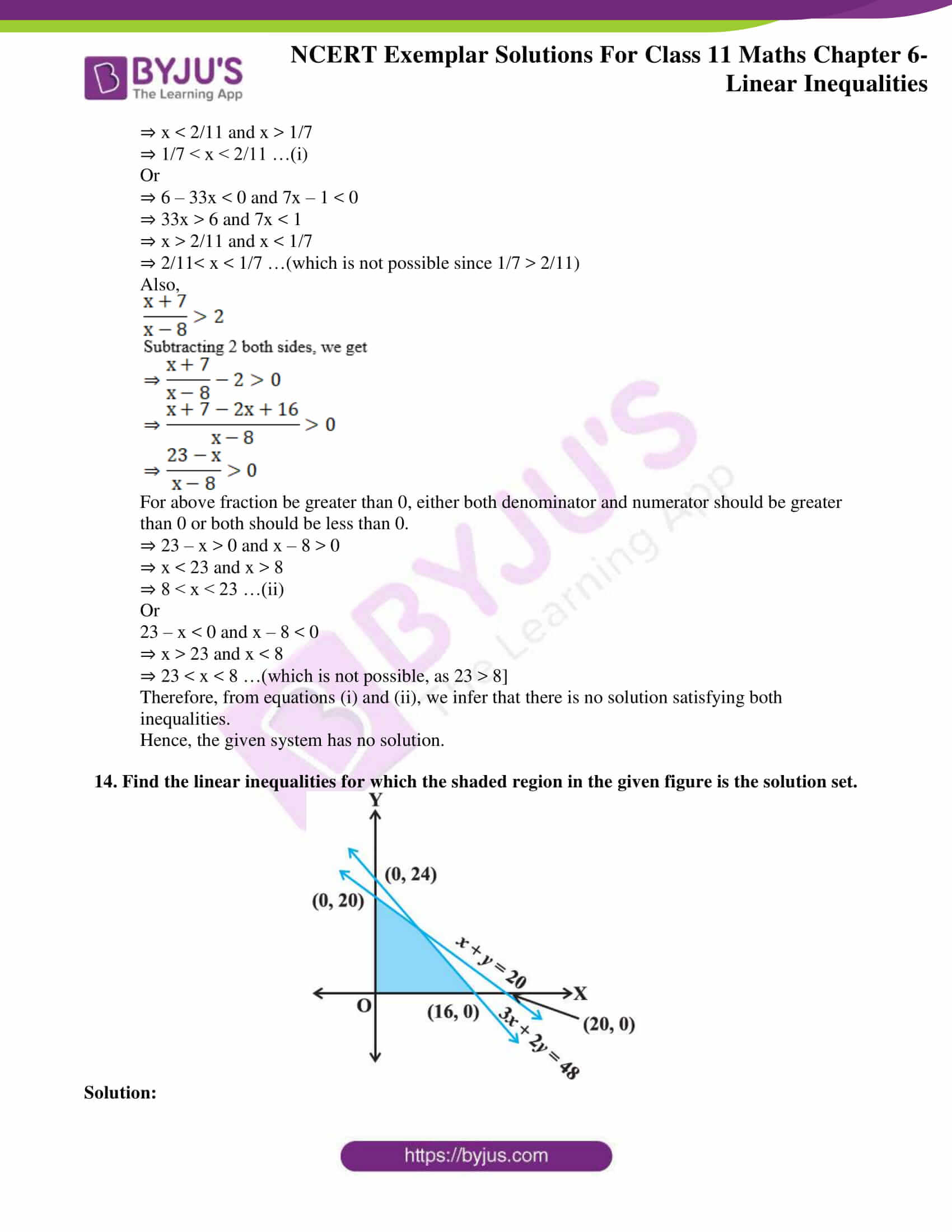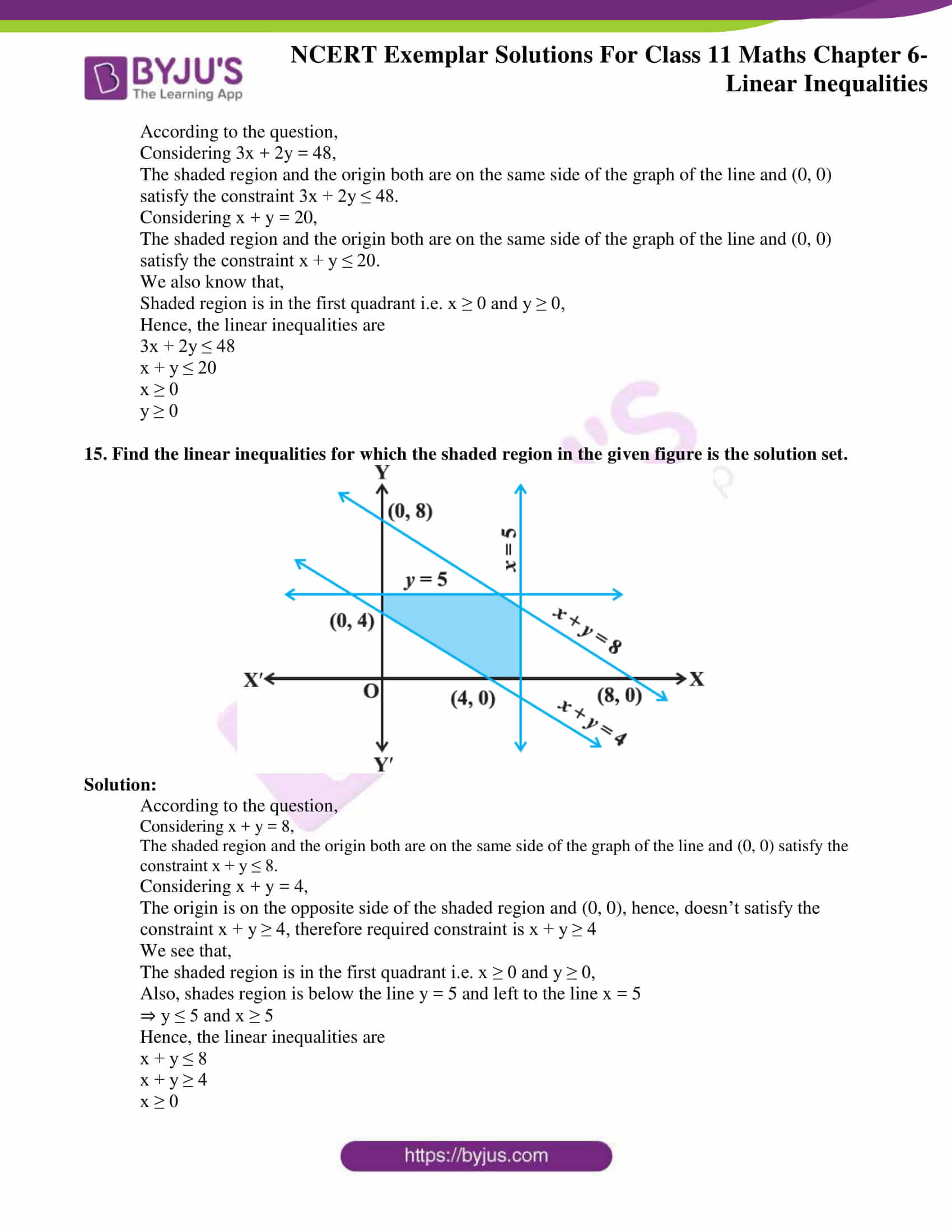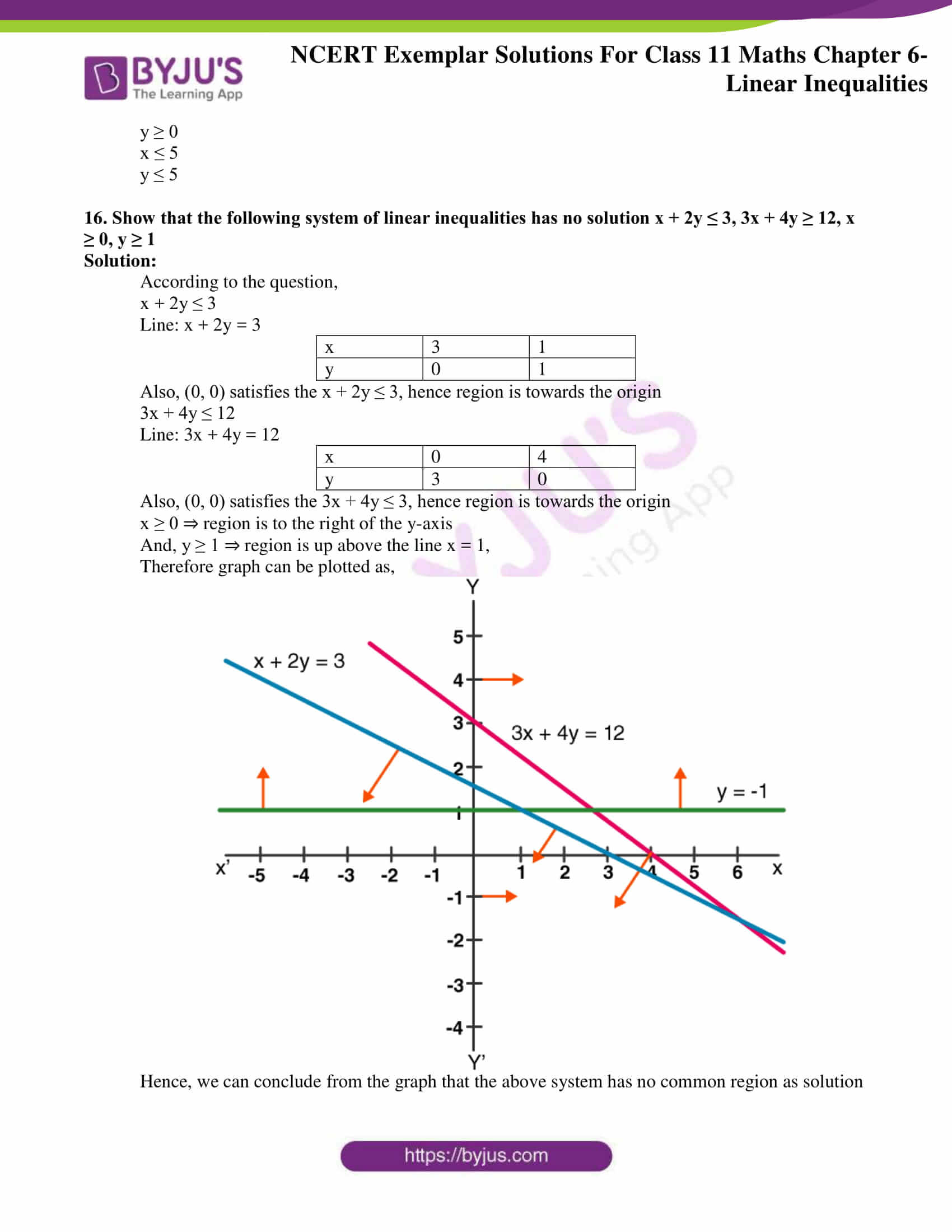### Access Answers to NCERT Exemplar Solutions for Class 11 Maths Chapter 6 Linear Inequalities

Exercise Page No: 107

Solve for x, the inequalities in Exercises 1 to 12.

1. Solve for x, the inequalities in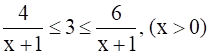Solution:

According to the question,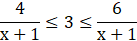Multiplying each term by (x + 1)

⇒ 4 ≤ 3(x + 1) ≤ 6

⇒ 4 ≤ 3x + 3 ≤ 6

Subtracting each term by 3, we get,

⇒ 1 ≤ 3x ≤ 3

Dividing each term by 3, we get,

⇒ (1/3) ≤ x ≤ 1

2. Solve for x, the inequalities in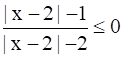Solution:

According to the question,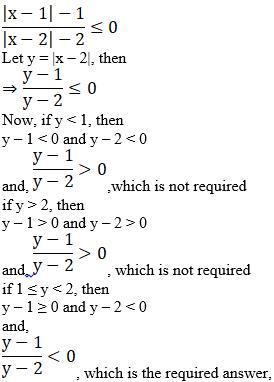Hence,

1 ≤ y < 2

⇒ 1 ≤ |x – 2| < 2

Here, there are two cases

⇒ 1 ≤ x – 2 < 2

⇒ 3 ≤ x < 4

And

⇒ 1 ≤ -(x – 2) < 2

⇒ 1 ≤ – x + 2 < 2

Multiplying each term by -1,

⇒ -2 ≤ x – 2 < -1

⇒ 0 ≤ x < 1

∴ Hence, the solution is [0, 1) ∪ [3, 4)

3. Solve for x, the inequalities in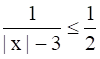Solution:

According to the question,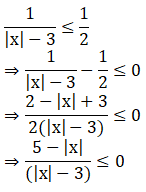⇒ 5 – |x| ≤ 0 and |x| – 3 > 0 or 5 – |x| ≥ 0 and |x| – 3 < 0

⇒ |x| ≥ 5 and |x| > 3 or |x| ≤ 5 and |x| < 3

⇒ |x| ≥ 5 or |x| < 3

⇒ x ∈ (- ∞ , – 5] or [5, ∞) or x ∈ ( -3 , 3)

⇒ x ∈ (- ∞ , – 5] ∪ ( -3, 3) ∪ [5, ∞)

4. Solve for x, the inequalities in |x – 1| ≤ 5, |x| ≥ 2

Solution:

|x – 1|≤ 5

There are two cases,

1:-

x – 1 ≤ 5

Adding 1 to LHS and RHS

⇒ x ≤ 6

2:-

⇒ -(x – 1) ≤ 5

⇒ -x + 1 ≤ 5

Subtracting 1 from LHS and RHS,

⇒ -x ≤ 4

⇒ x ≥ -4

From cases 1 and 2, we have

⇒ -4 ≤ x ≤ 6 …[i]

Also,

|x| ≥ 2

⇒ x ≥ 2 and

⇒ -x ≥ 2

⇒ x ≤ -2

⇒ x ∈ (∞, -2] ∪ [2, ∞) …[ii]

Combining equations [i] and [ii], we get

x ∈ [-4, -2] ∪ [2, 6]

5. Solve for x, the inequalities in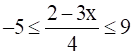Solution:

According to the question,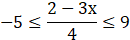Multiplying each term by 4, we get

⇒ -20 ≤ 2 – 3x ≤ 36

Adding -2 each term, we get

⇒ -22 ≤ -3x ≤ 34

Dividing each term by 3, we get

⇒ -22/3 ≤ -x ≤ 34/3

We know that,

Multiplication by -1 inverts the inequality.

So, multiplying each term by -1, we get

⇒  -34/3≤ x ≤ 22/3

6. Solve for x, the inequalities in 4x + 3 ≥ 2x + 17, 3x – 5 < – 2.

Solution:

According to the question,

4x + 3 ≥ 2x + 17

⇒ 4x – 2x ≥ 17 – 3

⇒ 2x ≥ 14

⇒ x ≥ 7 …(i)

Also,

3x – 5 < – 2

⇒ 3x < 3

⇒ x < 1 …(2)

Since, equations [i] and [ii] cannot be possible simultaneously,

We conclude that x has no solution.

7. A company manufactures cassettes. Its cost and revenue functions are C(x) = 26,000 + 30x and R(x) = 43x, respectively, where x is the number of cassettes produced and sold in a week. How many cassettes must be sold by the company to realise some profit?

Solution:

We know that,

Profit = Revenue – cost

The requirement is, profit > 0

According to the question,

Revenue, R(x) = 43 x

Cost, C(x) = 26,000 + 30 x; where x is number of cassettes

⇒ Profit = 43x – (26,000 + 30x) > 0

⇒ 13x – 26,000 > 0

⇒ 13x > 26000

⇒ x > 2000

Therefore, the company should sell more than 2000 cassettes to realise profit.

8. The water acidity in a pool is considered normal when the average pH reading of three daily measurements is between 8.2 and 8.5. If the first two pH readings are 8.48 and 8.35, find the range of pH value for the third reading that will result in the acidity level being normal.

Solution:

According to the question,

Now, let the third reading be ‘x’

Average pH should be between 8.2 and 8.5

Average pH = (8.48 + 8.35 + x)/3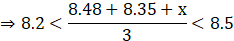Multiplying each term by 3, we get

⇒ 24.6 < 16.83 + x < 25.5

Subtracting 16.83 from each term, we get

⇒ 7.77 < x < 8.67

Therefore, from the above equation,

We get that,

The third pH reading should be between 7.77 and 8.67

9. A solution of 9% acid is to be diluted by adding 3% acid solution to it. The resulting mixture is to be more than 5% but less than 7% acid. If there is 460 litres of the 9% solution, how many litres of 3% solution will have to be added?

Solution:

According to the question,

Let x litres of 3% solution is to be added to 460 litres of the 9% of solution

Then, we get,

Total solution = (460 + x) litres

The total acid content in the resulting solution

= (460 × 9/100 + x × 3/100)

= (41.4 + 0.03x)%

According to the question, we have,

The resulting mixture should be more than 5% acidic but less than 7% acidic

So we get,

⇒ 5 % of (460 + x) < 41.4 + 0.03x < 7% of (460 + x)

⇒ 5/100 × (460 + x) < 41.4 + 0.03x < 7/100 × (460 + x)

⇒ 23 + 0.05 x < 41.4 + 0.03x < 32.2 + 0.07x

Now, we have

⇒ 23 + 0.05x < 41.4 + 0.03x and 41.4 + 0.03x < 32.2 + 0.07x

i.e., 0.02x < 18.4 and 0.04x > 9.2

⇒ 2x < 1840 and 4x > 920

⇒ x < 920 and x > 230

⇒ 230 < x < 920

Hence, solution between 230 l and 920 l should be added.

10. A solution is to be kept between 40°C and 45°C. What is the range of temperature in degree Fahrenheit, if the conversion formula is F = 9/5 C + 32?

Solution:

Let temperature in Celsius be C

Let temperature in Fahrenheit be F

According to the question,

Solution should be kept between 40° C and 45°C

⇒ 40 < C < 45

Multiplying each term by 9/5, we get

⇒ 72 < 9/5 C < 81

Adding 32 to each term, we get

⇒ 104 < 9/5 C + 32 < 113

⇒ 104 < F < 113

Hence, the range of temperature in Fahrenheit should be between 104° F and 113° F.

11. The longest side of a triangle is twice the shortest side and the third side is 2 cm longer than the shortest side. If the perimeter of the triangle is more than 166 cm then find the minimum length of the shortest side.

Solution:

Let the length of shortest side = ‘x’ cm

According to the question,

The longest side of a triangle is twice the shortest side

⇒ Length of largest side = 2x

Also, the third side is 2 cm longer than the shortest side

⇒ Length of third side = (x + 2) cm

Perimeter of triangle = sum of three sides

= x + 2x + x + 2

= 4x + 2 cm

Now, we know that,

Perimeter is more than 166 cm

⇒ 4x + 2 ≥ 166

⇒ 4x ≥ 164

⇒ x ≥ 41

Hence, minimum length of the shortest side should be = 41 cm.

12. In drilling world’s deepest hole, it was found that the temperature T in degree Celsius, x km below the earth’s surface was given by T = 30 + 25 (x – 3), 3 ≤ x ≤ 15. At what depth will the temperature be between 155°C and 205°C?

Solution:

According to the question,

T = 30 + 25(x – 3), 3 ≤ x ≤ 15; where, T = temperature and x = depth inside the earth

The Temperature should be between 155°C and 205°C,

So, we get,

⇒ 155 < T < 205

⇒ 155 < 30 + 25(x – 3) < 205

⇒ 155 < 30 + 25x – 75 < 205

⇒ 155 < 25x – 45 < 205

Adding 45 to each term, we get

⇒ 200 < 25x < 250

Dividing each term by 25, we get

⇒ 8 < x < 10

Hence, temperature varies from 155° C to 205° C at a depth of 8 km to 10 km.

13. Solve the following system of inequalities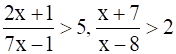Solution:

According to the question,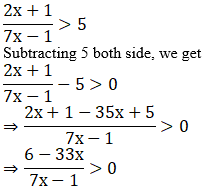For the above fraction be greater than 0, either both the denominator and numerator should be greater than 0 or both should be less than 0.

⇒ 6 – 33x > 0 and 7x – 1 > 0

⇒ 33x < 6 and 7x > 1

⇒ x < 2/11 and x > 1/7

⇒ 1/7 < x < 2/11 …(i)

Or

⇒ 6 – 33x < 0 and 7x – 1 < 0

⇒ 33x > 6 and 7x < 1

⇒ x > 2/11 and x < 1/7

⇒ 2/11< x < 1/7 …(which is not possible since 1/7 > 2/11)

Also,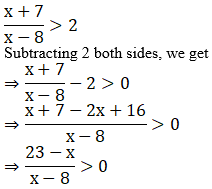For the above fraction be greater than 0, either both the denominator and numerator should be greater than 0 or both should be less than 0.

⇒ 23 – x > 0 and x – 8 > 0

⇒ x < 23 and x > 8

⇒ 8 < x < 23 …(ii)

Or

23 – x < 0 and x – 8 < 0

⇒ x > 23 and x < 8

⇒ 23 < x < 8 …(which is not possible, as 23 > 8]

Therefore, from equations (i) and (ii), we infer that there is no solution satisfying both inequalities.

Hence, the given system has no solution.

14. Find the linear inequalities for which the shaded region in the given figure is the solution set.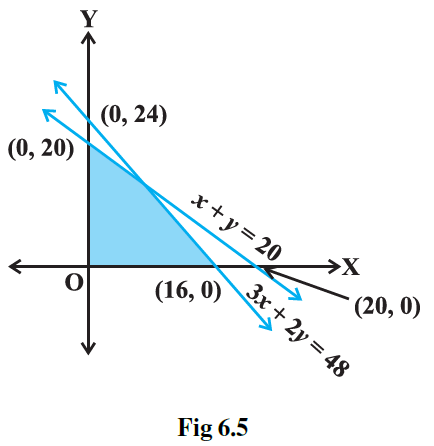Solution:

According to the question,

Considering 3x + 2y = 48,

The shaded region and the origin are both on the same side of the graph of the line, and (0, 0) satisfy the constraint 3x + 2y ≤ 48.

Considering x + y = 20,

The shaded region and the origin both are on the same side of the graph of the line, and (0, 0) satisfy the constraint x + y ≤ 20.

We also know that,

Shaded region is in the first quadrant i.e. x ≥ 0 and y ≥ 0,

Hence, the linear inequalities are

3x + 2y ≤ 48

x + y ≤ 20

x ≥ 0

y ≥ 0

15. Find the linear inequalities for which the shaded region in the given figure is the solution set.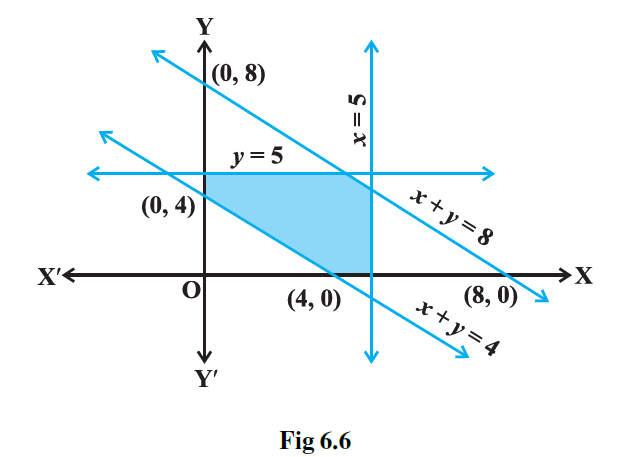Solution:

According to the question,

Considering x + y = 8,

The shaded region and the origin are both on the same side of the graph of the line, and (0, 0) satisfy the constraint x + y ≤ 8.

Considering x + y = 4,

The origin is on the opposite side of the shaded region, and (0, 0), hence, doesn’t satisfy the constraint x + y ≥ 4; therefore required constraint is x + y ≥ 4

We see that,

The shaded region is in the first quadrant i.e. x ≥ 0 and y ≥ 0,

Also, shades region is below the line y = 5 and left to the line x = 5

⇒ y ≤ 5 and x ≥ 5

Hence, the linear inequalities are

x + y ≤ 8

x + y ≥ 4

x ≥ 0

y ≥ 0

x ≤ 5

y ≤ 5

16. Show that the following system of linear inequalities has no solution x + 2y ≤ 3, 3x + 4y ≥ 12, x ≥ 0, y ≥ 1

Solution:

According to the question,

x + 2y ≤ 3

Line: x + 2y = 3

 x 3 1 y 0 1

Also, (0, 0) satisfies the x + 2y ≤ 3, hence region is towards the origin

3x + 4y ≤ 12

Line: 3x + 4y = 12

 x 0 4 y 3 0

Also, (0, 0) satisfies the 3x + 4y ≤ 3, hence region is towards the origin

x ≥ 0 ⇒ region is to the right of the y-axis

And, y ≥ 1 ⇒ region is up above the line x = 1,

Therefore graph can be plotted as,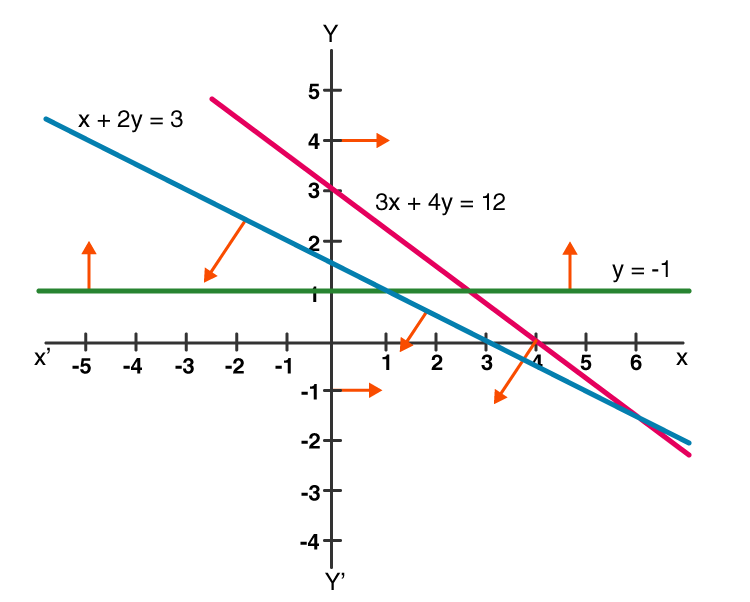Hence, we can conclude from the graph that the above system has no common region as a solution.